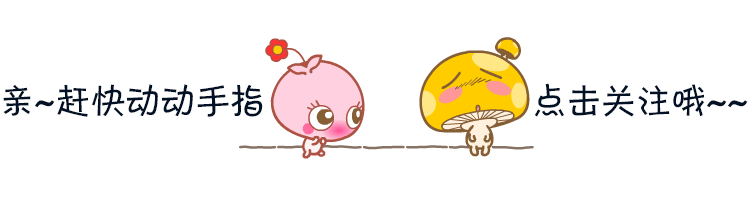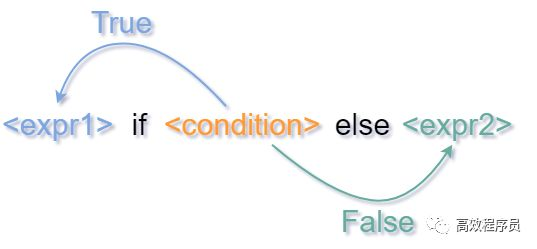# Python 条件表达式<expr1> if <condition> else <expr2>


1

# 常用方式

条件表达式的常见用途是变量的选择性赋值。

age = 12
if age < 18:
s = 'minor'
else:


s = 'minor' if age < 18 else 'adult'


2

# 优先级

x = 2
y = 5
z = 1 + x if x > y else y + 2
print(z)


z = (1 + x) if x > y else (y + 2)


z = 1 + (x if x > y else y) + 2


3

# 短路运算

和复合逻辑表达式一样，条件表达式也使用的短路运算。如果条件表达式的某些部分不需要计算，则不会进行计算。

• 如果 <condition> 为 True，则返回 <expr1><expr2> 不计算。

• 如果 <condition> 为 False，则返回 <expr2><expr1> 不计算。

x = 2 if True else 1/0

y = 1/0 if False else 5


4

# 替代 if/elif/else

条件表达式也可以链接在一起，作为一种替代 if/elif/else 的结构。

x = 2
if x == 1:
s = 'p'
elif x == 2:
s = 'y'
elif x == 3:
s = 't'
else:
s = 'h'


x = 2
s = ('p' if (x == 1) else
'y' if (x == 2) else
't' if (x == 3) else
'h'
)


s = ('p' if (x == 1) else
'y' if (x == 2) else
't' if (x == 3/0) else
'h'
)


·END·11-224966

#### python的输入输出及if判断语句

02-261万+

#### python条件表达式的四种实现方法02-052971

#### Python基础：条件表达式案例精选

10-252369

#### 需求：需要动态选择条件（参数）和操作符（加减乘除与或非）组成表达式。毫无头绪，请问有没有好的设计方案

04-09

04-28546

#### 第十一篇：赋值，表达式，print

02-201万+

#### php调试时echo,print_r(),var_dump()的区别

08-175646

#### Python基本输出函数print()用法小结

11-2230

#### python简单学------------python基础、print,input,if判断等

02-18101

#### 为什么print在Python 3中变成了函数？©️2020 CSDN 皮肤主题: 技术黑板 设计师: CSDN官方博客点击重新获取扫码支付1.余额是钱包充值的虚拟货币，按照1:1的比例进行支付金额的抵扣。
2.余额无法直接购买下载，可以购买VIP、C币套餐、付费专栏及课程。余额充值